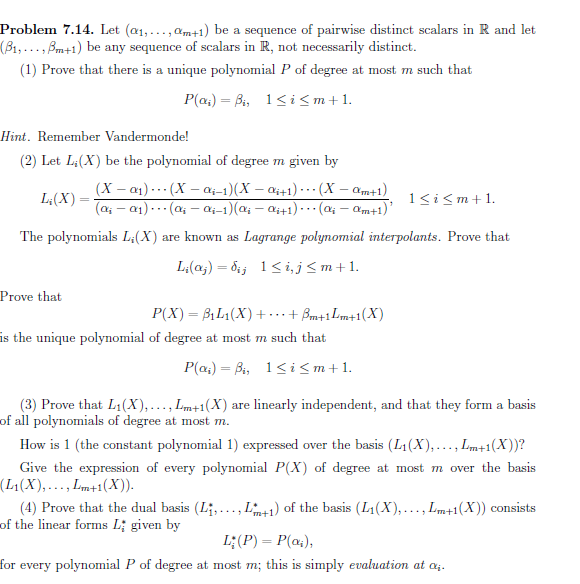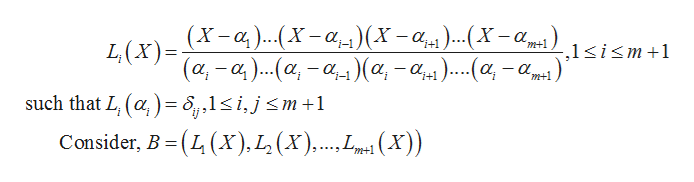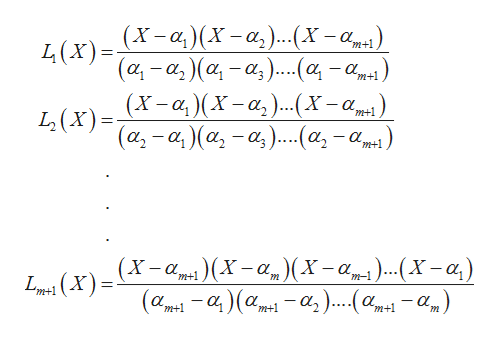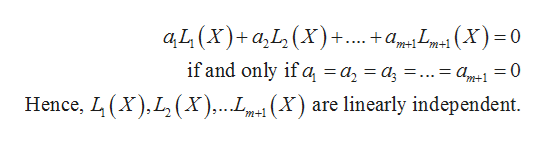Problem 7.14. Let (æ1,...,am+1) be a sequence of pairwise distinct scalars in R and let(B1,...,Bm+1) be any sequence of scalars in R, not necessarily distinct.(1) Prove that there is a unique polynomial P of degree at most m such thatP(a4) Bi, 1i

Question

Hello, kindly assist me with the solution to Q3. I will appreciate it if you provide a very detailed solution, thankshelp_outlineImage TranscriptioncloseProblem 7.14. Let (æ1,...,am+1) be a sequence of pairwise distinct scalars in R and let (B1,...,Bm+1) be any sequence of scalars in R, not necessarily distinct. (1) Prove that there is a unique polynomial P of degree at most m such that P(a4) Bi, 1i
Step 1

Given thathelp_outlineImage Transcriptionclose(X-a..(X -a_^)(X-a)...(X -a, L (x)= ( α,-α). . α-α, )(α, - α,μ)...(α -αμ.). i+1 m+1 -,1si
Step 2

Then the values arehelp_outlineImage TranscriptioncloseL(x)-X-a)(X-a,)...(X-a LX)-α-αα-α)...(ατα.. m+1 _ m+1 (X-a)X-a,)(x-a L(X)=a,-a, )(a,-a).(a,-) m+1 m+1 -a(X-a (X-a)...Xa,) X -а, _ m-1 m+1 m 1(X) -α-α α.α, )... (α.αμ) m+1 -a) m+1 m+1 m+1 m fullscreen
Step 3

Since, these values can not be written as lin...help_outlineImage TranscriptioncloseaL(x)+a,L (X)+.... +amX)=0 m+1m+1 if and only if a = a2 = a3 =. Pm+1 (XL (x),. (X) are linearly independent Hence, m+1 fullscreen

Want to see the full answer?

See Solution

Want to see this answer and more?

Our solutions are written by experts, many with advanced degrees, and available 24/7

See Solution
Tagged in

Math